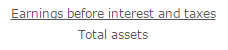Accounting

# What is Return on Total Assets?

The return on total assets compares the earnings of a business to the total assets invested in it. The measure is intended to discern whether management can effectively utilize assets to generate a reasonable return.

The calculation of the return on total assets is earnings before interest and taxes (EBIT), divided by the total assets figure listed on the balance sheet. The EBIT figure is used instead of net profits in order to focus attention on operating earnings.

The formula is:For example, ABC International reports a net income of \$100,000. This figure includes interest expense of \$12,000 and taxes of \$28,000. When these two expenses are added back, the EBIT of the company is \$140,000. The total assets figure for the company is \$4,000,000. Therefore, the return on total assets is:

\$140,000 EBIT / \$4,000,000 Total assets = 3.5% Return on total assets

The total assets figure is inclusive of contra accounts, which means that accumulated depreciation and the allowance for doubtful accounts are subtracted from the gross amount of assets on the balance sheet.

The concept is useful for comparison purposes. For example, an outside analyst can compare the return on total assets of a number of competitors in the same industry to determine which one is reporting the most efficient asset usage in comparison to earnings.

Internally, the concept can be used as the basis for a detailed investigation of which assets are nonproductive and so should be disposed of. It can also lead to an examination of the working capital investment, to see if operating policies can be adjusted to minimize the amount of working capital.

A concern with this measurement is that the denominator is derived from book values, rather than market values. This is of particular concern when a business has a large investment in fixed assets that have a higher value than is indicated by their reported book values. In this case, the calculated return on total assets is higher than is really the case, since the denominator is too low.

Another concern with this measurement is that it does not focus on how assets were financed. If a business used high-cost debt to buy its assets, the return on total assets could be favorable, while the business is actually at risk of defaulting on the associated interest expense.